# 人工智能实践：Tensrfow笔记

##### 一. 人工智能概述

###### 2. 人工智能、机器学习、深度学习之间的关系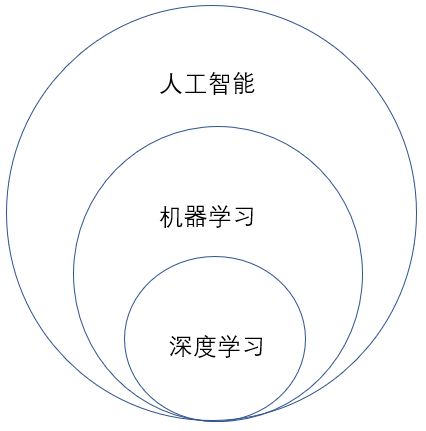###### 4. 机器学习与传统机器工作的差异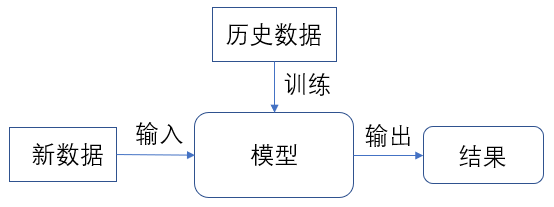###### 9. 神经元模型是什么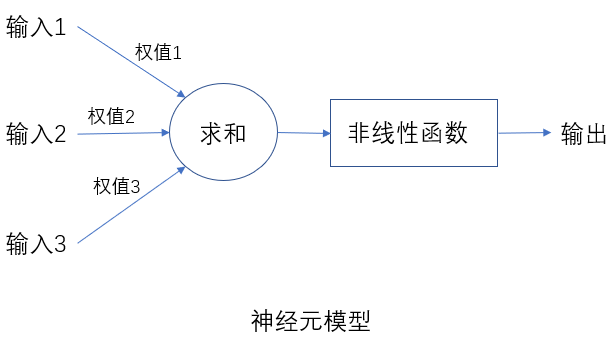###### 10. 计算机神经网络的发展（三起两落）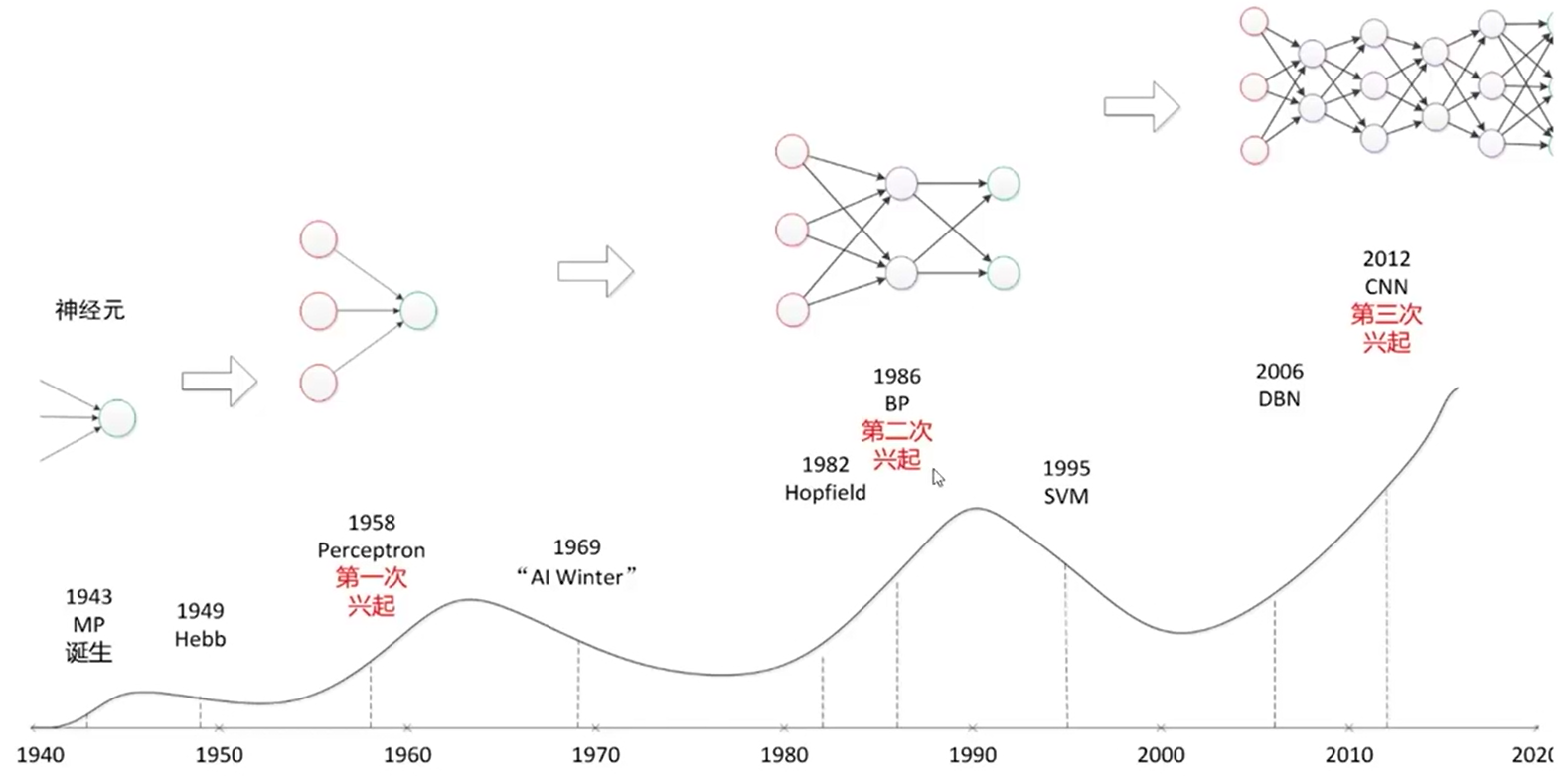##### 二. Tensorflow框架
###### 1. 神经网络的参数

W = tf.Variable(tf.random_normal([2,3],stddev=2,mean=0,seed=1))

tf.random_normal()：生成正太分布的随机数

[2,3]：产生2行3列矩阵

stddev=2：标准差为2

mean=0：均值为0。随机种子如果去掉，每次生成的随机数会不一致

seed=1：随机种子为1

tf.random_normal()函数可以用tf.truncated_normal()函数替换，表示去掉过大偏离点的正太分布，如果随机生成的数据偏离平均值超过两个标准差，这个数据将重新生成。用平均分布函数tf.random_uniform()替换。

tf.zeros生成全0数组：tf.zeros([3,2],int(32))生成[[0,0],[0,0],[0,0]]

tf.ones生成全1数组：tf.ones([3,2],iint(32))生成[[1,1],[1,1],[1,1]]

tf.fill生成全定值数组：tf.fill([3,2],6)生成[[6,6],[6,6],[6,6]]

tf.constant直接给出数组的值，tf.contant([3,2,1])生成[3,2,1]

###### 2. 神经网络的实现过程

1、准备数据集，提取特征，作为输入，喂给神经网络（NN）
2、搭建NN结构，从输入到输出（先搭建计算图，再用会话执行）（NN前向传播算法，计算输出）
3、大量数据喂给NN，迭代优化NN参数（NN反向传播算法，优化参数训练模型）
4、使训练好的模型预测分类

###### 3. 搭建第一个神经网络
• 基于Tensorflow的NN
用张量表示数据，用计算图搭建神经网络，用会话执行计算图，优化线上的权重（参数），得到模型。

• 张量（tensor）
张良就是多维数组（列表），张量可以表示0阶到n阶数组（列表）。用阶表示张量的维数，0阶张量就是标量，表示一个单独的数。

0-D 0 标量scalar s = 123
1-D 1 向量vector v = [1,2,3]
2-D 2 矩阵matrix m = [[1,2,3],[,4,5,6],[7,8,9]]
n-D n 张量tensor t = [[[…
• 修改vim

[root@instance-mtfsf05r ~]# vim ~/.vimrc，在.vimrc文件中写入：

set ts = 4
ser nm


ts = 4表示在vim中缩进一个tab键缩进4个空格
nm在vim中显示行号

• 两个张量的加法
# coding:utf-8
# 实现两个张量的加法
import tensorflow as tf  # 导入tensorflow模块，简写为tf

a = tf.constant([1.0, 2.0])  # 定义张量a,constant方法用来定义常数
b = tf.constant([3.0, 4.0])  # 定义张量b
rs = a + b
print(rs)


add:0：表示张量的名字

add：节点

0：第0个输出

shape=(2,)：维度信息

shape：维度

(2，)：一维数组，长度为2

dtype=float32：表示数据类型

dtype：数据类型

float32：浮点型数据

• 计算图（Graph）

y = XW = x1 * w1 + x2 * w2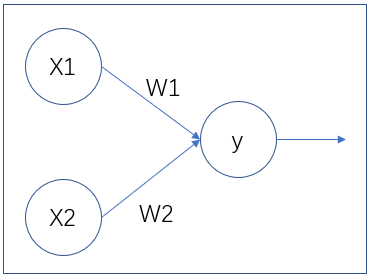# coding:utf-8
# 实现两个张量的加法
import tensorflow as tf  # 导入tensorflow模块，简写为tf

x = tf.constant([[1.0, 2.0]])  # 定义张量a,constant方法用来定义常数
w = tf.constant([[3.0], [4.0]])  # 定义张量b
y = tf.matmul(x, w)
print(y)


• 会话（Session）

# coding:utf-8
# 实现两个张量的加法
import tensorflow as tf  # 导入tensorflow模块，简写为tf

x = tf.constant([[1.0, 2.0]])  # 定义张量a,constant方法用来定义常数
w = tf.constant([[3.0], [4.0]])  # 定义张量b
y = tf.matmul(x, w)
# print(y)
# 使用Session得到运算结果
with tf.Session() as sess:
print(sess.run(y))  # [[11.]]


###### 4. 前向传播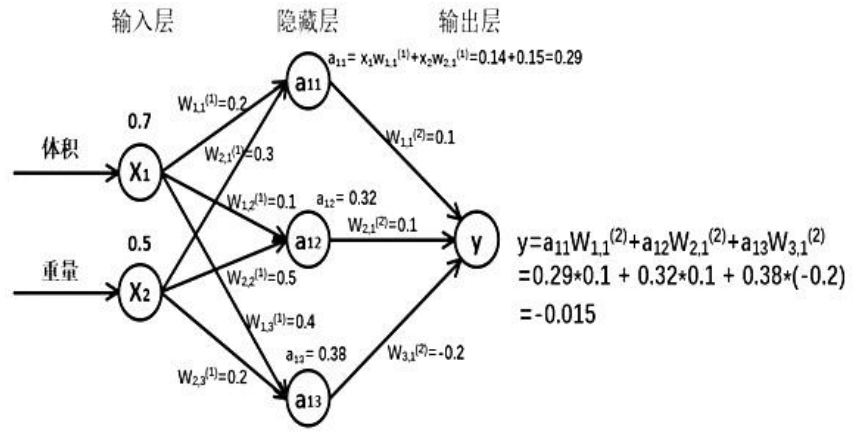Tensorflow描述前向传播：

X是输入为1x2的矩阵，W前节点编号，后节点编号（层数）为待优化的参数。

W(1)为2x3的矩阵：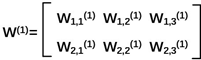a(1) = [a11,a12,a13]为1x3的矩阵=XW(1)
W(2)是3x1的矩阵：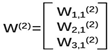y = a(1)W(2)

a = tf.matmul(X,W1)
y = tf.matmul(a,W2)

# coding:utf-8
# 两层简单神经网络(1)
import tensorflow as tf

# 定义输入和参数
x = tf.constant([[0.5, 0.8]])
w1 = tf.Variable(tf.random_normal([2, 3], stddev=1, seed=1))
w2 = tf.Variable(tf.random_normal([3, 1], stddev=1, seed=1))
# 定义前向传播的过程
a = tf.matmul(x, w1)
y = tf.matmul(a, w2)
# 用会话计算结果
with tf.Session() as sess:
init_op = tf.global_variables_initializer()  # 初始化所有变量的函数简写为初始化节点，用init_op表示
sess.run(init_op)
print(sess.run(y))  # [[3.1674924]]


# coding:utf-8
# 两层简单神经网络
import tensorflow as tf

# 定义输入和参数
# 使用placeholder方法实现输入定义，可以在with结构中喂入多组输入特征,方便批量处理
x = tf.placeholder(tf.float32, shape=(1, 2))
w1 = tf.Variable(tf.random_normal([2, 3], stddev=1, seed=1))
w2 = tf.Variable(tf.random_normal([3, 1], stddev=1, seed=1))
# 定义前向传播的过程
a = tf.matmul(x, w1)
y = tf.matmul(a, w2)
# 用会话计算结果
with tf.Session() as sess:
init_op = tf.global_variables_initializer()  # 初始化所有变量
sess.run(init_op)
# 喂入一组特征
print(sess.run(y, feed_dict={x: [[0.5, 0.8]]}))  # [[3.1674924]]


# coding:utf-8
# 两层简单神经网络
import tensorflow as tf

# 定义输入和参数
# 使用placeholder方法实现输入定义，可以在with结构中喂入多组输入特征,方便批量处理.session.run喂入多组数据
x = tf.placeholder(tf.float32, shape=(None, 2))
w1 = tf.Variable(tf.random_normal([2, 3], stddev=1, seed=1))
w2 = tf.Variable(tf.random_normal([3, 1], stddev=1, seed=1))
# 定义前向传播的过程
a = tf.matmul(x, w1)
y = tf.matmul(a, w2)
# 用会话计算结果
with tf.Session() as sess:
init_op = tf.global_variables_initializer()  # 初始化所有变量
sess.run(init_op)
# 喂入一组特征
print(sess.run(y, feed_dict={x: [[0.5, 0.8], [0.1, 0.2], [0.3, 0.4], [0.3, 0.6], [0.2, 0.9]]}))  # [[3.1674924]]
print()
print("w1:", sess.run(w1))
print()
print('w2:', sess.run(w2))
'''
[[3.1674924 ]
[0.72020966]
[1.7270732 ]
[2.160629  ]
[2.5243084 ]]

w1: [[-0.8113182   1.4845988   0.06532937]
[-2.4427042   0.0992484   0.5912243 ]]

w2: [[-0.8113182 ]
[ 1.4845988 ]
[ 0.06532937]]
'''